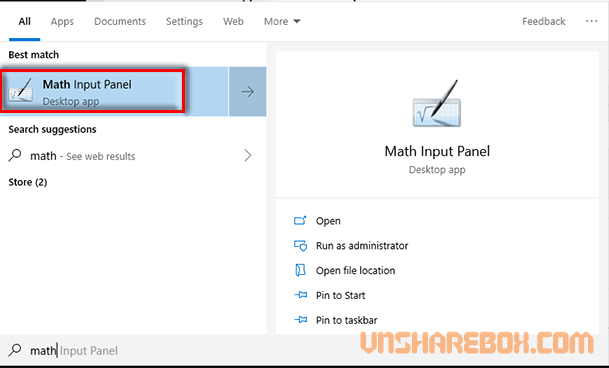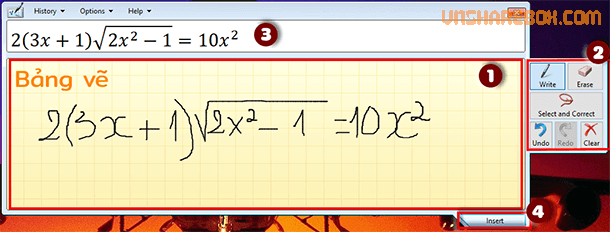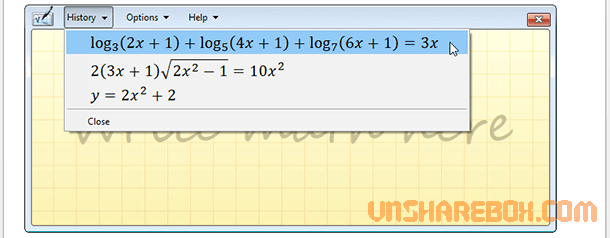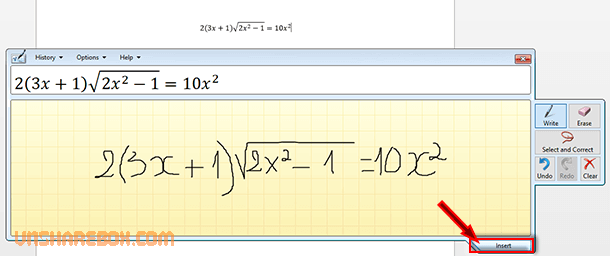Home Office Simple way to create math equations in MS Word

# Simple way to create math equations in MS Word

If you used Equation to create math equations in Word, you will learn one more easier way through this article.

Different from using Equation patterns available in Word. This uses the drawing tool, so you can easily create new custom equations that Equation in Microsoft Word is not available. The tool is the Math Input Panel and it is preinstalled on Windows 10.

## How to draw math equations using Math Input Panel on Windows 10

To open the Math Input Panel, open Start Windows and you can use search key “math” to quick find Math Input Panel.In the Math Input Panel interface, you can use the mouse to draw on the Drawing Board (1) and automatically identify equation will appear in the preview box (3). If the drawing process fails, you can use tool (2) like:

• Write: Drawing mode.
• Erase: Delete selected.
• Select and Correct: help you choose equation are composing and automatically propose reasonable equations.
• Undo: return to 1 operation.
• Clear: Delete the Drawing board.After creating an equation, click Insert (4) to save and you can view it again in History.And to add the equation created with Math Input Panel into MS Word then you need to open Word and place the cursor in Word > at the Math Input Panel, select the equation are editing or from History > select Insert is done.So, you have learned one more way to create math equations in Word. This way is quite easy to implement and suitable for creating equations if Word does not support it.

Have fun!